### C. Wirth, J. W. Lichstein, J. Dushoff, A. Chen, and F. S. Chapin, III. 2008. White spruce meets black spruce: dispersal, postfire establishment, and growth in a warming climate. Ecological Monographs 78:489–505.

Appendix E. Derivation of negative binomial distribution for the number of recruits.

We assume that seed rain (S) comes from a negative binomial distribution with parameters q (probability of success) and r (shape), so that the probability of s seeds arriving is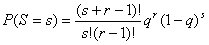with mean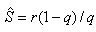and variance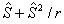(Casella and Berger 2002). We assume that each seed has a probability p of establishing and surviving, so that the number of recruits (N) comes from a binomial distribution with s trials and probability of success p . The conditional probability of n seeds recruiting given seed rain s is then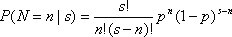The unconditional, or marginal, probability of n seeds recruiting is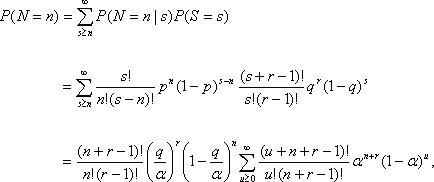where us - n and α ≡ 1 - (1 - p)(1 - q). The summand is a negative binomial probability, so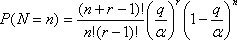which is negative binomial with mean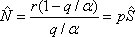and variance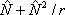.

LITERATURE CITED

Casella, G., and R. L. Berger. 2002. Statistical Inference. Second Edition. Duxbury Press. 688 pp.

[Back to M078-019]